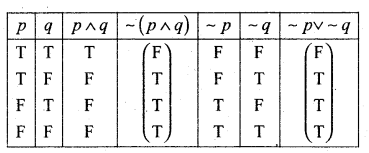# 2nd PUC Basic Maths Question Bank Chapter 6 Mathematical Logic

Students can Download Basic Maths Question Bank Chapter 6 Mathematical Logic Questions and Answers, Notes Pdf, 2nd PUC Basic Maths Question Bank with Answers helps you to revise the complete Karnataka State Board Syllabus and to clear all their doubts, score well in final exams.

## Karnataka 2nd PUC Basic Maths Question Bank Chapter 6 Mathematical Logic

### 2nd PUC Basic Maths Mathematical Logic One Mark Questions and Answers

Question 1.
Write the verbal form of the following compound proposition given:
p: x is a real number
q: Mathematics is easy
r: Today is a holiday

• (p ∨ q) = x is a real number or mathematics is easy
• (~p ∧ r) = x is not a real number and maths is easy
• (q ∧ ~ p) = Maths is easy and today is not a holiday
• (p ↔ q) ∨ = r x is a real number if and only if mathematics is easy or today
is a holiday
• ((p ∨ q) → q) =  If x is a real number or today is a holiday then maths is easy
• (p ∨ r) ∧ ~ q = If x is a real number or today is a holiday then mathematics is
not easy.

Question 2.
Symbolise the following propositions.
(i) 3x = 9 and x < 7
Let p : 3x = 9, q : x < 1
The given proposition in symbols is p ∧ q

(ii) 33 ÷ − 11 ≠ 3 or 8 – 6 = 2
= ~ p or q ⇒~p v q(iii) If oxygen is a gas then gold is a compound
p → q

(iv) y + 4 ≠ 4 or e is not a vowel
~ pv ~ q

(v) If p :  $$\sqrt{3}$$ is an integer
q : 2 is an odd number
r : 5 is a prime number.

Question 3.
What is the truth values of the following?

(i) (p ↔ q) ∧ r
Here p = F, q – F, r = T
(F↔ F) ∧ T = T ∧ T = T
∴ Given pro position is true.

(ii) (p → q)∧ ~ r
(F → F) ∧ ~ T = T ∧ F = F

(iii) (p ∧ q) → r
(F ∧ F) ⇒ T = F → T = T(iv) (~p ∨ q) ∧  r
(~F ∨ F) ∧ T = (T ∨ F) ∧ T = T ∧ T = T

Question 4.
Negate the following.
(i) p ∨ ~q
~(p ∨ ~q) ≡ ~p ∧ ~ (~q) = ~ p ∧ q

(ii) (~ p → q)
– (~ p → q) =~ P∧ ~ q                   ,

(iii) ~ p ∧ ~q
~ (~ p∧ ~ q) = p ∨ q

(iv) p∧ ~ q
~(p∧ ~ q)=~ p ∨ q

(v) 4 is an even integer or 7 is a prime number.
Given = p ∨ q
~(p ∨ q) ≡ ~ p ∧ ~ q
4 is not an even integer and 7 is not a prime number.

(vi) He likes to run and he does not like to sit.
He does not likes to run or he likes to sit.(vii) He likes mathematics and he does not like logic.
He does not like mathematics or he likes logic.

(viii) If two triangles are similar then their areas are equal.
Given (p → q)
~(p → q) ≡ p∧ ~ q
Two triangles are similar and their areas are not equal.

### 2nd PUC Basic Maths Mathematical Logic Two Marks Questions and Answers

Question 1.
If p, q and r are propositions with truth values F, T, and F respectively, then find the truth values of the following compound propositions.

(i) (~ p → q) ∨ r
(~ F → T) ∨ F
≡ (T→ T) ∨ F ≡ T ∨ F = T

(ii) (p ∧ ~ q) →  r
(F∧~T) → F
(F∧F) → F ≡ F → F ≡ T(iii) P→ (q → r)
F → (T→ F)
F→ (F) = T

(iv) ~{p~>q)v ~(p ↔ q)
~(F → T) ∨ – (F↔T)
~(T) ∨ ~(F)
14 is not a divisor of 48 or 28 is divisible by 82.

### 2nd PUC Basic Maths Mathematical Logic Three Marks Questions and Answers

Question 1.
If the compound proposition p → (q ∨ r) is False.
Then find the truth values of p, q and r.
Given p → (q ∨ r) is False
⇒ p is true and (q v r) is. False
p is true and ⇒ q is False and r is False
∴ p = T,q = F,r = F.

Question 2.
If the truth value of the proposition (p ∧ q) → (r ∨ ~ s) is false then find the truth val­ues of p, q, r and s.
Given: (p ∧ q) → (r ∨ ~ s) is False
T → F = F
∴ p ∧ q is true and r v~ s is False
⇒ (p is true and q is true) and (r is false and ∼ s is false)
⇒ p = T, q = T, r = F, s = T.

Question 3.
Construct the truth table for the following
(i) (~ pA ~q)

 p q ~ p ~q ~ pA ~q T T F F F T F F T F F T T F F F F T T T

(ii) ~(p → q)

 p q p → q ~(p → q) T T T F T F F T F T T F F F T F(iii) (p ∨ q) ∧ ∼ p

 p q p∨q ~p (p∨q) ∧~p T T T F F T F T F F F T T T T F F F T F

(iv) (p ∨ q) ↔ (q ∧ p)

 p q p∨q q∧p (p∨q)↔ (q~p) T T T T T T F T F F F T T F F F F F F T

(v) ∼ p (p∧∼q)

 p q ∼p ∼q p∧∼q ∼p ↔ (p∧∼q) T F F F F T T F F T T F F T T F F F F F T T F F

Question 4.
Write the converse, Inverse and contrapositive of the following,
(i) If x ∈ A ∩ B then x ∈ A and x ∈ B
Given in symbols = p →(q ∧ r)
Converse = (q ∧ r) → p
If x ∈ A & x ∈ B Then x ∈ A∩B
Inverse =~ p → ~ (q ∧ r)
~ p → (~q∨ ~ r)
If x∉A∩B then x ∉ q or x ∉ B
Contrapositive =~ (q ∧ r) → ~ p
If x∈ A or x∉B Then x∉ A∩B(ii) If the questions are easy then students score better marks.
Converse:- If students score better marks then the questions are easy.
Inverse:- If questions are not easy then student do not score better marks. Contrapositive:- If the students do not score better marks then the questions are not easy.

(iii) If x2 =y2 then x = y.
Convers = If x = y then x2= y2
Inverse = If x2 ≠ y2 then x ≠ y
Contrapositive = If x ≠ y then x2 ≠ y2

### 2nd PUC Basic Maths Mathematical Logic Five Marks Questions and Answers

I. Question 1.
Construct the truth tables for the following.
(i) p ∨(q ∧ ∼r)

 p q r ∼ r q∧∼ r p∨(q∧~r) T T T F F T T T F T T T T F T F F T T F F T F T F T T F F F F T F T T T F F T F F F F F F T F F

(ii) (∼p ∧ q) ∧ ∼ r

 p q r ~r ~p ~p∧q (~p∧q)∧~r T T T F F F F T T F T F F F T F T F F F F T F F T F F F F T T F T T F F T F T T T T F F T F T F F F F F T T F F(iii) (p∧q) →(r ∨ ∼ q)

 p q r ~q p∧q rv ~ q p∧q → rv ~ q T T T F T T T T T F F T F F T F T T F T T T F F T F T T F T T F F T T F T F F F F T F F T T F T T F F F T F T T

(iv) (p∧q) ∨ r

 P q r (p∧q) (p∧q)∧r T T T T T T T F T T T F T F T T F F F F F T T F T F T F F F F F T F T F F F F F

(v) (p → q) →  ~ r

 p q r p → q ~r (p → q)→ ~r T T T T F F T T F T T T T F T F F T T F F F T F F T T T F F F T F T T T F F T T F F

(vi) (p → r) ∧( p→ q)

 p q r p → r p →q (p → r) a (p → q) T T T T T T T T F F T F T F T T F F T F F F F F F T T T T T F T F T T T F F T T T T F F F T T TII. Verify the following for Tautology, Contadiction or neither.

Question 1.
(~ p∨q) ∨ (P∧~q)

 p q ~P ~q ~p∨q p∧~q (~p∨q)v(p∧~q) T T F F T F T T F F T F T T F T T F T F T F F T T T F T

From last colum we conclude that it is a tautology.

Question 2.
(p∨~q) ∧ {~ p∨q)

 p q ~q p∧~q ~p q∨ ~p (p∧~q)∧(~p∨q) T T F F F T F T F T T F F F F T F F T T F F F T F T T F

From last column we conclude that it is a contradiction.

Question 3.
(p → q) ∨ (~p→ q)

 p q p → q ~p ~p →q (p → q) ∨ (~ p→q) T T T F T T T F F F T T F T T T T T F F T T F T

From last column we conclude that it is a Tautology.Question 4.
(p → q) ↔ (~p ∨ q)

 p q p → q ~p ~p ∨ q (p → q)↔(∼ p ∨ q) T T T F T T T F F F F T F T T T T T F F T T T T

From last column we conclude that it is a Tautology.

Question 5.
[(p∧q) ∨ (p∨q) ]↔ q

 p q p∧q p∨q {p∧q)∨(p∨q) [(p∧q)∨(p∨q)]↔q T T T T T T T F F T T F F T F T T T F F F F F T

From last colum we canclude that it is neither Tautology nor Contradiction.

Question 6.
P.T. (p → q) ↔  (~ q → p) is a Tautology.

 p q p→q ~q ~P ~q→~p (p → q) (~ q→~p) T T T F F T T T F F T F F T F T T F T T T F F T T T T T

From last colum we conclude that it is a Tautology

Question 7.
P. T. [(P → q) ∧ (q→ r)→ (p →R) is a TautologyFrom last colum we canclude that it is a Tautology.Question 8.
(∼p∧q) →(p∨q)

 p q ~p ~p∧q p∨q (p∧q) → (p∨q) T T F F T T T F F F T T F T T T T T F F T F F T

Question 9.
Verify [p ∧ (p ∨ q)] → q is a Tautology or contradiction.

 p q ~ p (p∨q) ~p∧(p∨q) [~p∨(p∨q)] →  q T T F T F T T F F T F T F T T T T T F F T F F T

From last colum we canclude that it is a Tautology.

Question 10.
Verify (p → – q) ∨ (~ p ↔ q) for a Tautology or not.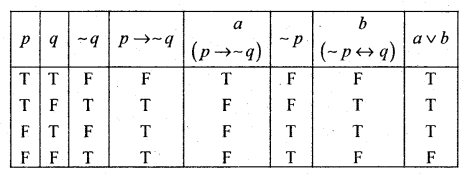From last colum we conclude that it is neither Tautology nor contradiction.

III. Examine whether the following are logically equivalent?

Question 1.
p ↔ q and (p → q) ∧ (q → p)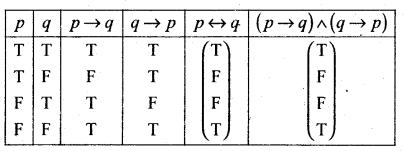From 5th and 6th colums we get (p ↔ q) ≡ (p → q) a (q → p)

Question 2.
p → (q → r) and (p → q) → rQuestion 3.
(p ∧~q) ∨ q and p ∨ q.Question 4.
p ∧ q and ~ (p → ~ q)Question 5.
~(p ↔ q) and (p∧~q) ∨ (q∧p)From 5th and 8th colum we conclude ~(p ↔ q) ≡ (p∨ ~ q) ∨(q∧~p)Question 6.
p ↔ q an (∼p∨q ) ∧ (~ q v p)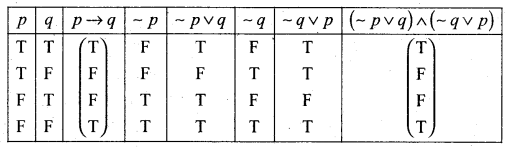From 3rd and 8th colums we conclude that (p ↔ q) = (~ p ∨ q) ∧ (~q ∨ p)

Question 7.
S.T. ~(p ∼ q) ≡ ∼p ∧- q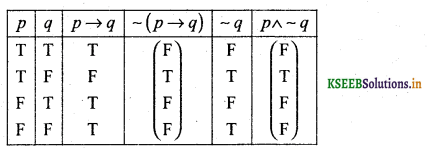From 4th and 6th colums we get ~ (p → q) ≡ p∧ ∼ q

Question 8.
S.T. ~(p ∧ q) ≡ ~ pv ~ q